SSC CGL Previous Year Questions: Data Interpretation - 5

# SSC CGL Previous Year Questions: Data Interpretation - 5 - SSC CGL

Test Description

## 39 Questions MCQ Test SSC CGL (Tier - 1) - Previous Year Papers (Topic Wise) - SSC CGL Previous Year Questions: Data Interpretation - 5

SSC CGL Previous Year Questions: Data Interpretation - 5 for SSC CGL 2023 is part of SSC CGL (Tier - 1) - Previous Year Papers (Topic Wise) preparation. The SSC CGL Previous Year Questions: Data Interpretation - 5 questions and answers have been prepared according to the SSC CGL exam syllabus.The SSC CGL Previous Year Questions: Data Interpretation - 5 MCQs are made for SSC CGL 2023 Exam. Find important definitions, questions, notes, meanings, examples, exercises, MCQs and online tests for SSC CGL Previous Year Questions: Data Interpretation - 5 below.
Solutions of SSC CGL Previous Year Questions: Data Interpretation - 5 questions in English are available as part of our SSC CGL (Tier - 1) - Previous Year Papers (Topic Wise) for SSC CGL & SSC CGL Previous Year Questions: Data Interpretation - 5 solutions in Hindi for SSC CGL (Tier - 1) - Previous Year Papers (Topic Wise) course. Download more important topics, notes, lectures and mock test series for SSC CGL Exam by signing up for free. Attempt SSC CGL Previous Year Questions: Data Interpretation - 5 | 39 questions in 40 minutes | Mock test for SSC CGL preparation | Free important questions MCQ to study SSC CGL (Tier - 1) - Previous Year Papers (Topic Wise) for SSC CGL Exam | Download free PDF with solutions
 1 Crore+ students have signed up on EduRev. Have you?
SSC CGL Previous Year Questions: Data Interpretation - 5 - Question 1

### DIRECTIONS: The sub divided bar diagram given below depicts H.S. Students of a school for three years. Study the diagram and answer the questions.        (SSC CGL 1st Sit. 2013)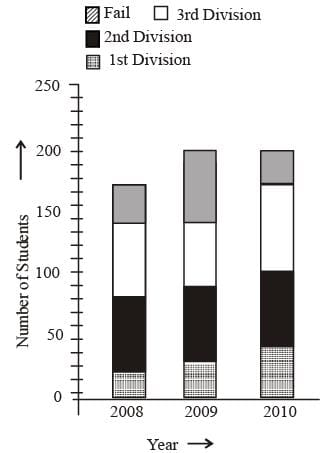Q. In which year the school had the best result for H.S. in respect of percentage of pass candidates?

Detailed Solution for SSC CGL Previous Year Questions: Data Interpretation - 5 - Question 1

By observing the graph, we can say that percentage of the best result in 2010.

SSC CGL Previous Year Questions: Data Interpretation - 5 - Question 2

### DIRECTIONS: The sub divided bar diagram given below depicts H.S. Students of a school for three years. Study the diagram and answer the questions.        (SSC CGL 1st Sit. 2013)Q. The number of students passed in third division in the year 2008 was

Detailed Solution for SSC CGL Previous Year Questions: Data Interpretation - 5 - Question 2

Total number of students pased in third division in the year 2008 = 60

SSC CGL Previous Year Questions: Data Interpretation - 5 - Question 3

### DIRECTIONS: The sub divided bar diagram given below depicts H.S. Students of a school for three years. Study the diagram and answer the questions.        (SSC CGL 1st Sit. 2013)Q. The percentage of the students passed in 2nd division in the year 2010 was

Detailed Solution for SSC CGL Previous Year Questions: Data Interpretation - 5 - Question 3

Total students passed in 2nd division in 2010 = 60 total students in 2010 = 200
∴ Required percentage = (60/200)x100 = 30%.

SSC CGL Previous Year Questions: Data Interpretation - 5 - Question 4

DIRECTIONS: The adjacent histogram shows the average pocket money received by 60 students for a span of one month. Study the diagram and answer the question.       (SSC CGL 1st Sit. 2013)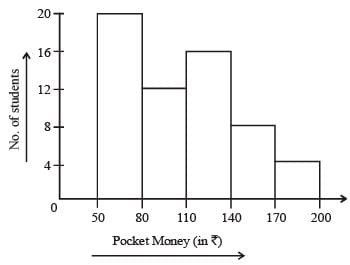Q. The number of students who received pocket money upto ₹ 140 is

Detailed Solution for SSC CGL Previous Year Questions: Data Interpretation - 5 - Question 4

Required answer = 20 + 12 + 16 = 48

SSC CGL Previous Year Questions: Data Interpretation - 5 - Question 5

DIRECTIONS: The pass percentage for an examination in a school is shown in the adjoining bar diagram, for males and females separately for four years. Study the diagram and answer the question.        (SSC CGL 1st Sit. 2013)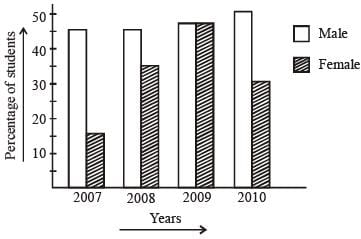Q. The maximum percentage of students passed in the year is

Detailed Solution for SSC CGL Previous Year Questions: Data Interpretation - 5 - Question 5

By observing the graph, we can say that yr. 2009 has maximum percentage of students passed in the year.

SSC CGL Previous Year Questions: Data Interpretation - 5 - Question 6

DIRECTIONS: The pass percentage for an examination in a school is shown in the adjoining bar diagram, for males and females separately for four years. Study the diagram and answer the question.        (SSC CGL 1st Sit. 2013)Q. The year in which the difference of pass percentage between male and female is maximum, is

Detailed Solution for SSC CGL Previous Year Questions: Data Interpretation - 5 - Question 6

By observing the graph, we can say that difference of pass percentage between male and female is maximum in 2007.

SSC CGL Previous Year Questions: Data Interpretation - 5 - Question 7

DIRECTIONS: The following graph shows the expenditure incurred in bringing a book, by a magazine producer. Study the graph and answer question.        (SSC CGL 1st Sit. 2013)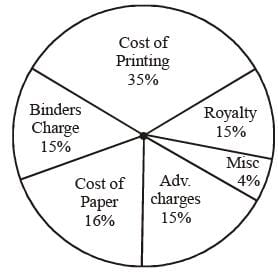Q. What should be the central angle of the sector for the cost of the paper?

Detailed Solution for SSC CGL Previous Year Questions: Data Interpretation - 5 - Question 7

Central angle of the sector for cost of the paper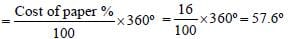SSC CGL Previous Year Questions: Data Interpretation - 5 - Question 8

DIRECTIONS: The following graph shows the expenditure incurred in bringing a book, by a magazine producer. Study the graph and answer question.        (SSC CGL 1st Sit. 2013)Q. If the miscellaneous charges are ₹ 6,000, the cost of paper is

Detailed Solution for SSC CGL Previous Year Questions: Data Interpretation - 5 - Question 8

If the cost of paper = ₹ x, then from the given pie-chart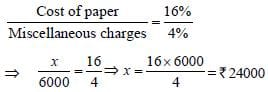SSC CGL Previous Year Questions: Data Interpretation - 5 - Question 9

DIRECTIONS: The following graph shows the expenditure incurred in bringing a book, by a magazine producer. Study the graph and answer question.        (SSC CGL 1st Sit. 2013)Q. If 5500 copies are published, miscellaneous expenditures amount to ₹ 1,848, find the cost price of 1 copy.

Detailed Solution for SSC CGL Previous Year Questions: Data Interpretation - 5 - Question 9

Given,
Miscellaneous expenditure = 1848
⇒ 4% of the total expenditure cost for publishing 5500 copies = 1848
⇒ Total expenditure cost of 5500 copies (i.e. 100%)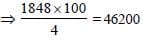∴ Expenditure cost per copy = 46200/5500 = 8.40
∴ So, marked price of each copy = 8.40 + 25% of 8.40
= 8.40 + 2.10 = 10.50

SSC CGL Previous Year Questions: Data Interpretation - 5 - Question 10

DIRECTIONS: The following pie-chart shows the marks scored by a student in different subject - viz. Physics (Ph), Chemistry (Ch), Mathematical (M), Social Science (SS) and English (E) in an examination. Assuming that total marks obtained for the examination in 810, answer the questions given below.        (SSC CGL 1st Sit. 2013)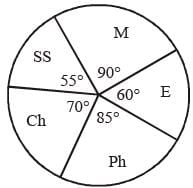Q. The subject in which the student obtained 135 marks is

Detailed Solution for SSC CGL Previous Year Questions: Data Interpretation - 5 - Question 10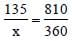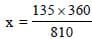⇒ 60° which is in english.

SSC CGL Previous Year Questions: Data Interpretation - 5 - Question 11

DIRECTIONS: The following pie-chart shows the marks scored by a student in different subject - viz. Physics (Ph), Chemistry (Ch), Mathematical (M), Social Science (SS) and English (E) in an examination. Assuming that total marks obtained for the examination in 810, answer the questions given below.        (SSC CGL 1st Sit. 2013)Q. The marks obtained in English, Physics and Social Science exceed the marks obtained in Mathematics and Chemistry by

Detailed Solution for SSC CGL Previous Year Questions: Data Interpretation - 5 - Question 11

English + Physics + Social Science = 200°
Maths + Chemistry = 160°
Required percentage
= (40/160) x 100 = 25%

SSC CGL Previous Year Questions: Data Interpretation - 5 - Question 12

DIRECTIONS: The following pie-chart shows the marks scored by a student in different subject - viz. Physics (Ph), Chemistry (Ch), Mathematical (M), Social Science (SS) and English (E) in an examination. Assuming that total marks obtained for the examination in 810, answer the questions given below.        (SSC CGL 1st Sit. 2013)Q. The difference of marks between Physics and Chemistry is same as that between

Detailed Solution for SSC CGL Previous Year Questions: Data Interpretation - 5 - Question 12

Difference of corresponding angles :
Physics and Chemistry = 85 – 70 = 15°
Chemistry and Social science = 70 – 55 = 15°

SSC CGL Previous Year Questions: Data Interpretation - 5 - Question 13

DIRECTIONS: The following pie-chart shows the marks scored by a student in different subject - viz. Physics (Ph), Chemistry (Ch), Mathematical (M), Social Science (SS) and English (E) in an examination. Assuming that total marks obtained for the examination in 810, answer the questions given below.        (SSC CGL 1st Sit. 2013)Q. The marks obtained in Mathematics and Chemistry exceed the marks obtained in Physics and Social Science by

Detailed Solution for SSC CGL Previous Year Questions: Data Interpretation - 5 - Question 13

Sum of corresponding angles of Maths and Chemistry
= 90 + 70 = 160°
Sum of corresponding angles of Physics and Social Science
= 85 + 55 = 140°
Difference = 20°
∵360° ≡ 810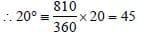SSC CGL Previous Year Questions: Data Interpretation - 5 - Question 14

DIRECTIONS: Shown below is the multiple bar diagram depicting the changes in the roll strength of a college in four faculties from 2001 – 02 to 2003 – 04.            (SSC CGL 1st Sit.2013)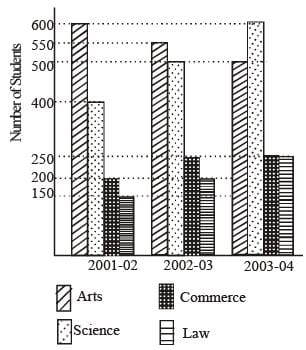Q. The percentage of students in Science faculty in 2001 – 2002 is

Detailed Solution for SSC CGL Previous Year Questions: Data Interpretation - 5 - Question 14

Total students in 2001 – 2002 = 1350
∴ Required percentage = (400/1350)x100 = 29.6

SSC CGL Previous Year Questions: Data Interpretation - 5 - Question 15

DIRECTIONS: Shown below is the multiple bar diagram depicting the changes in the roll strength of a college in four faculties from 2001 – 02 to 2003 – 04.            (SSC CGL 1st Sit.2013)Q. The percentage of students in Law faculty in 2003 – 04 is

Detailed Solution for SSC CGL Previous Year Questions: Data Interpretation - 5 - Question 15

Total students in 2003 – 04 = 1600
∴ Required percentage
= (250/1600)x100 = 15.6%.

SSC CGL Previous Year Questions: Data Interpretation - 5 - Question 16

DIRECTIONS: Shown below is the multiple bar diagram depicting the changes in the roll strength of a college in four faculties from 2001 – 02 to 2003 – 04.            (SSC CGL 1st Sit.2013)Q. Percentage of increase in Science students in 2003 – 04 over 2001 – 2002 is

Detailed Solution for SSC CGL Previous Year Questions: Data Interpretation - 5 - Question 16

Required percentage increase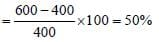SSC CGL Previous Year Questions: Data Interpretation - 5 - Question 17

DIRECTIONS: Study the following histogram and answer the following.       (SSC CGL 2nd Sit.2013)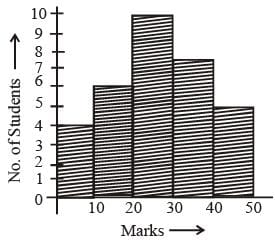Q. The total number of studen ts in volved in the data is

Detailed Solution for SSC CGL Previous Year Questions: Data Interpretation - 5 - Question 17

Required number of students = 4 + 6 + 10 + 8 + 5 = 33.

SSC CGL Previous Year Questions: Data Interpretation - 5 - Question 18

DIRECTIONS: Study the following histogram and answer the following.       (SSC CGL 2nd Sit.2013)Q. The maximum number of students got the marks in the interval of

Detailed Solution for SSC CGL Previous Year Questions: Data Interpretation - 5 - Question 18

The maximum number of students got the mark in the internal
⇒ (20 – 30) = 10

SSC CGL Previous Year Questions: Data Interpretation - 5 - Question 19

DIRECTIONS: Study the following histogram and answer the following.       (SSC CGL 2nd Sit.2013)Q. The least number of students got the marks in the interval

Detailed Solution for SSC CGL Previous Year Questions: Data Interpretation - 5 - Question 19

The minimum number of students got the mark in the interval
⇒ (0 – 10) = 4

SSC CGL Previous Year Questions: Data Interpretation - 5 - Question 20

DIRECTIONS: Study the following histogram and answer the following.       (SSC CGL 2nd Sit.2013)Q. The ratio of the students obtaining marks in the first and the last interval is

Detailed Solution for SSC CGL Previous Year Questions: Data Interpretation - 5 - Question 20

Required ratio = 4 : 5

SSC CGL Previous Year Questions: Data Interpretation - 5 - Question 21

DIRECTIONS: Study the following histogram and answer the following.       (SSC CGL 2nd Sit.2013)Q. In a certain country, allocations to various sectors of the yearly budget per ₹ 1000 crores are represented by this piediagram. The expenditure (in ₹) on Agriculture is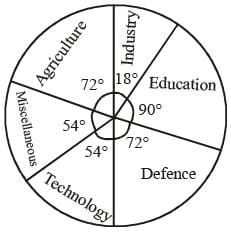Detailed Solution for SSC CGL Previous Year Questions: Data Interpretation - 5 - Question 21

Expenditure on agriculture
= (72/360) x 1000
₹ 200 crore

SSC CGL Previous Year Questions: Data Interpretation - 5 - Question 22

Study the above bar graph showing the production of food grains (in million tons).       (SSC CGL 2nd Sit.2013)
What is the ratio between the maximum production and the minimum production during the given period?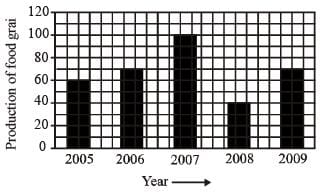Detailed Solution for SSC CGL Previous Year Questions: Data Interpretation - 5 - Question 22

Required ratio = 100 : 40 = 5 : 2

SSC CGL Previous Year Questions: Data Interpretation - 5 - Question 23

DIRECTIONS: Study the two pie-charts and answer the questions.       (SSC CGL 1st Sit. 2013)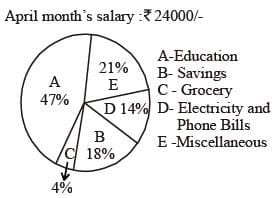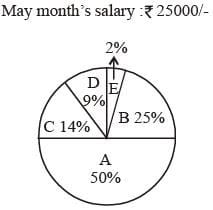Q. The average amount spent on Education, Grocery and Savings from April month's salary is :

Detailed Solution for SSC CGL Previous Year Questions: Data Interpretation - 5 - Question 23

Required average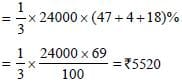SSC CGL Previous Year Questions: Data Interpretation - 5 - Question 24

DIRECTIONS: Study the two pie-charts and answer the questions.       (SSC CGL 1st Sit. 2013)Q. From the salary of May, the amount spent on Grocery and Electricity are :

Detailed Solution for SSC CGL Previous Year Questions: Data Interpretation - 5 - Question 24

Expenditure on grocery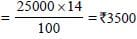Expenditure on electricty =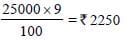SSC CGL Previous Year Questions: Data Interpretation - 5 - Question 25

DIRECTIONS: Study the two pie-charts and answer the questions.       (SSC CGL 1st Sit. 2013)Q. The ratio of amount spent for savings in April month's salary and miscellaneous in May month's salary is :

Detailed Solution for SSC CGL Previous Year Questions: Data Interpretation - 5 - Question 25

Required ratio= 24 × 18 : 25 × 2 = 216 : 25

SSC CGL Previous Year Questions: Data Interpretation - 5 - Question 26

DIRECTIONS: Study the two pie-charts and answer the questions.       (SSC CGL 1st Sit. 2013)Q. What is the percent increase in Education in May month than April month ?

Detailed Solution for SSC CGL Previous Year Questions: Data Interpretation - 5 - Question 26

Expenditure on education in April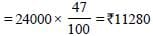Expenditure on education in May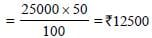Percentage increase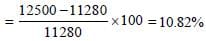SSC CGL Previous Year Questions: Data Interpretation - 5 - Question 27

DIRECTIONS: The following pie-chart shows the number of students admitted in different faculties of a college. Study the chart and answer the question.        (SSC CGL 1st Sit.2013)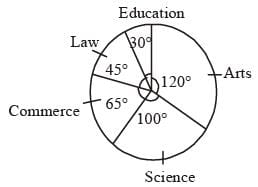Q. If 1000 students are admitted in science, what is the total number of students?

Detailed Solution for SSC CGL Previous Year Questions: Data Interpretation - 5 - Question 27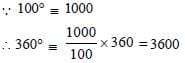SSC CGL Previous Year Questions: Data Interpretation - 5 - Question 28

DIRECTIONS: The following pie-chart shows the number of students admitted in different faculties of a college. Study the chart and answer the question.        (SSC CGL 1st Sit.2013)Q. If 1000 students are admitted in science, what is the ratio of students in science and arts?

Detailed Solution for SSC CGL Previous Year Questions: Data Interpretation - 5 - Question 28

Required ratio = 100 : 120 = 5 : 6

SSC CGL Previous Year Questions: Data Interpretation - 5 - Question 29

DIRECTIONS: The following pie-chart shows the number of students admitted in different faculties of a college. Study the chart and answer the question.        (SSC CGL 1st Sit.2013)Q. How many students are more in commerce than in law if 1000 students are in science?

Detailed Solution for SSC CGL Previous Year Questions: Data Interpretation - 5 - Question 29

Difference between the angles of students of commerce and science
∵ 100° = 1000
∴ 1° = 10
Difference between commerce and law = (65° – 45°) = 20°
∴ 20° = 200

SSC CGL Previous Year Questions: Data Interpretation - 5 - Question 30

DIRECTIONS: Production of three different flavours soft drinks X, Y and Z for a period of six years has been expressed in the following graph. Study the graph and answer the questions.       (SSC Sub. Ins. 2013)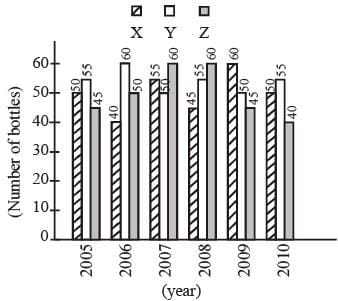Q. The approximate decline in the production of flavour Z in 2010 as compared to the production in 2008 is:

Detailed Solution for SSC CGL Previous Year Questions: Data Interpretation - 5 - Question 30

Percentage decrease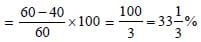SSC CGL Previous Year Questions: Data Interpretation - 5 - Question 31

DIRECTIONS: Production of three different flavours soft drinks X, Y and Z for a period of six years has been expressed in the following graph. Study the graph and answer the questions.       (SSC Sub. Ins. 2013)Q. The average annual production was maximum in the given period for the flavour:

Detailed Solution for SSC CGL Previous Year Questions: Data Interpretation - 5 - Question 31

Average annual production:
Flavour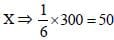lakh bottles
Flavour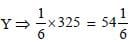lakh bottles
Flavour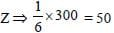lakh bottles

SSC CGL Previous Year Questions: Data Interpretation - 5 - Question 32

DIRECTIONS: Production of three different flavours soft drinks X, Y and Z for a period of six years has been expressed in the following graph. Study the graph and answer the questions.       (SSC Sub. Ins. 2013)Q. What percent of the total production of flavour X in 2005 and 2006 combined is the total production of flavour Z in 2007 and 2008 combined?

Detailed Solution for SSC CGL Previous Year Questions: Data Interpretation - 5 - Question 32

Total production of flavour X in 2005 and 2006 = 90
Total production of flower Z in 2007 and 2008 = 120.
Required percentage
= (120/90) x 100 = 133.3

SSC CGL Previous Year Questions: Data Interpretation - 5 - Question 33

DIRECTIONS: Production of three different flavours soft drinks X, Y and Z for a period of six years has been expressed in the following graph. Study the graph and answer the questions.       (SSC Sub. Ins. 2013)Q. The percentage of rise/fall in production from the previous year is maximum for the flavour Y in this year:

Detailed Solution for SSC CGL Previous Year Questions: Data Interpretation - 5 - Question 33

Percentage increase/decrease: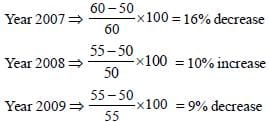SSC CGL Previous Year Questions: Data Interpretation - 5 - Question 34

DIRECTIONS: Production of three different flavours soft drinks X, Y and Z for a period of six years has been expressed in the following graph. Study the graph and answer the questions.       (SSC Sub. Ins. 2013)Q. The difference (in lakh bottles) between the average production of flavour X in 2005, 2006, 2007 and the average production of flavour Y in 2008, 2009 and 2010 is :

Detailed Solution for SSC CGL Previous Year Questions: Data Interpretation - 5 - Question 34

Required difference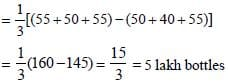SSC CGL Previous Year Questions: Data Interpretation - 5 - Question 35

DIRECTIONS: The following table shows the productions of food-grains (in million tons) in a state for the period 1999 - 2000 to 2003 - 2004. Read the table and answer the questions.          (SSC Sub. Ins. 2013)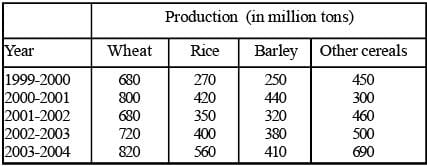Q. In 2002 - 2003, the percentage increase in the production of barley as compared to the previous year was:

Detailed Solution for SSC CGL Previous Year Questions: Data Interpretation - 5 - Question 35

Percent increase =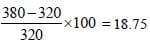SSC CGL Previous Year Questions: Data Interpretation - 5 - Question 36

DIRECTIONS: The following table shows the productions of food-grains (in million tons) in a state for the period 1999 - 2000 to 2003 - 2004. Read the table and answer the questions.          (SSC Sub. Ins. 2013)Q. During the period 1999 - 2000 to 2003 - 2004, x percent of the total production is production of wheat. The value of x is about:

Detailed Solution for SSC CGL Previous Year Questions: Data Interpretation - 5 - Question 36

Total production:
Wheat ⇒ 3700 million tonnes
Rice ⇒ 2000 million tonnes
Barley ⇒ 1800 million tonnes
Other cereals ⇒ 2400 million tonnes
Required answer =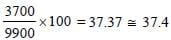SSC CGL Previous Year Questions: Data Interpretation - 5 - Question 37

DIRECTIONS: The following table shows the productions of food-grains (in million tons) in a state for the period 1999 - 2000 to 2003 - 2004. Read the table and answer the questions.          (SSC Sub. Ins. 2013)Q. In the year 2003 - 2004, the increase in production was maximum over the previous year for:

Detailed Solution for SSC CGL Previous Year Questions: Data Interpretation - 5 - Question 37

Percentage increase: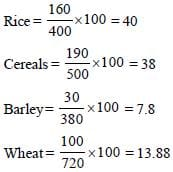SSC CGL Previous Year Questions: Data Interpretation - 5 - Question 38

DIRECTIONS: The following table shows the productions of food-grains (in million tons) in a state for the period 1999 - 2000 to 2003 - 2004. Read the table and answer the questions.          (SSC Sub. Ins. 2013)Q. The difference of average production of rice and the average production of barley over the years is :

Detailed Solution for SSC CGL Previous Year Questions: Data Interpretation - 5 - Question 38

Required difference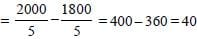million tonnes

SSC CGL Previous Year Questions: Data Interpretation - 5 - Question 39

Different choices made by a group of 200 students are given below in percentage. The number of students who have taken neither Science nor Commerce is       (SSC Multitasking 2013)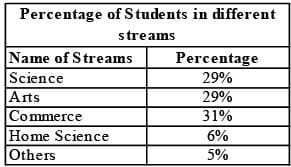Detailed Solution for SSC CGL Previous Year Questions: Data Interpretation - 5 - Question 39

Students having both science and commerce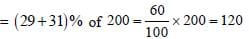Students who have taken neither science nor commerce = Total students – Students having both scicence and commerce
= 200 –120 = 80

## SSC CGL (Tier - 1) - Previous Year Papers (Topic Wise)

250 tests
Information about SSC CGL Previous Year Questions: Data Interpretation - 5 Page
In this test you can find the Exam questions for SSC CGL Previous Year Questions: Data Interpretation - 5 solved & explained in the simplest way possible. Besides giving Questions and answers for SSC CGL Previous Year Questions: Data Interpretation - 5, EduRev gives you an ample number of Online tests for practice

250 tests